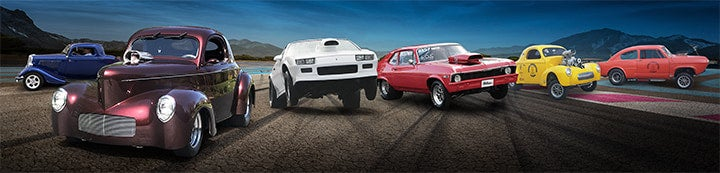1 - 3 of 3 Posts

#### Sir Joab

·
##### MOPAR Guy
Joined
·
186 Posts
Discussion Starter
To start with, I'm not a math-a-maniac, but I do like to use mathematics to predict the outcome of things I do. Here's my question: you could increase an engine's torque by increasing the bore, but you also could increase the torque by increasing the stroke. So, in light of this, would it be correct to say that:

torque increase (roughly) = displacement increase

If you calculate it in terms of PSI in the cylinder this equation is correct.

Am I on the right track?#### xntrik

·
##### Save a horse, Ride a Cowboy.
Joined
·
5,122 Posts
Sir Joab said:
To start with, I'm not a math-a-maniac, but I do like to use mathematics to predict the outcome of things I do. Here's my question: you could increase an engine's torque by increasing the bore, but you also could increase the torque by increasing the stroke. So, in light of this, would it be correct to say that:

torque increase (roughly) = displacement increase

If you calculate it in terms of PSI in the cylinder this equation is correct.

Am I on the right track?Well, yes, sort of. Let's avoid semantics.
K I S S

Torque increases with

1) more surface to push on = larger bore with same psi
2) longer lever to push = longer stroke with same psi
3) increased dynamic compression ratio = more psi
4) supercharging =higher oxygen content for greater burn= more psi
5) nitrous oxide =higher oxygen content for greater burn= more psi

6) you could also consider less internal friction

#### techinspector1

·
##### Registered
Joined
·
17,612 Posts
Yeah, what xntrik said.

1 - 3 of 3 Posts